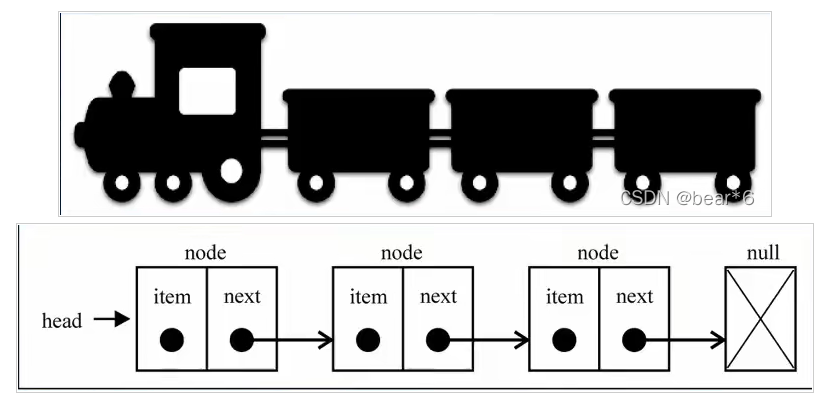﻿ JavaScript实现单链表过程解析_javascript技巧_脚本之家
javascript技巧# JavaScript实现单链表过程解析

• 数组的创建通常要申请一段连续的内存空间，并且大小是固定的，所以当当前数组不能满足容量需求时，需要进行扩容，（一般是申请一个更大的数组，然后将原数组中的元素复制过去）
• 在数组元素开头或者中间位置插入数据的成本很高，需要进行大量元素的位移。

• 内存空间不必是连续的，可以充分利用计算机的内存，实现灵活的内存动态管理。
• 链表不必在创建时就确定大小，并且大小可以无限延伸下去。
• 链表在插入和删除数据的时候，事件复杂度可以达到O（1），相对数组效率高很多。

• 链表访问任何一个位置的元素的时候，都需要从头开始访问，无法通过下标直接访问元素。

## 一、什么是单链表## 二、单链表的封装

``` function LinkedList(){
this.length = 0;
//内部的类
function Node(data){
this.data = data;
this.next = null;
}
}

```

## 三、单链表的常用操作

• `append（element) `:向列表尾部添加一个项
• `insert(position,element)` :向列表的特定位置插入一个项
• `get(position)` :获取对应位置的元素
• `indexOf(element)` :返回元素在列表中的索引，如果列表中没有该元素则返回-1
• `update(position，ele)` :修改某个位置的元素
• `removeAt(position)` :从列表的指定位置移除一项
• `remove(element) `:从列表中移除一项
• `isEmpty()` :如果链表中不包含任何元素，返回true,如果链表长度大于0返回false
• `size()` :返回链表包含的元素个数，与数组的`length`属性相关
• `toString() `:由于列表项用到了Node类，需要重写继承自`JavaScript`对象默认的toString方法，让其输出元素的值

### 1、append(element)方法-----向列表尾部添加一个项

• 链表本身为空，新添加的数据是唯一的节点
• 链表不为空，需要向其他节点后面追加节点

```LinkedList.prototype.append = function(data){
var newNode = new Node(data);
// 判断链表是否为空
// 1.为空
if(this.length === 0){
}else{
//不为空
if(current.next){
current = current.next;
}
current.next = newNode;
}
this.length += 1;
}

```

### 2、toString方法-----输出元素的值

```LinkedList.prototype.toString = function(){
var ListStr = '';
while(current){
ListStr += current.data + ' ';
current = current.next;
}
return ListStr;
}

```

``` var list = new LinkedList();
list.append('a');
list.append('b');
list.append('c');
list.append('d');
list.append('e');

```### 3、insert方法-----向列表的特定位置插入一个项

``` LinkedList.prototype.insert = function(position,data){
if(position<0 || position >this.length){
return false;
}
var newNode = new Node(data);
var index = 0;
if(position == 0){

}else{
while(index++ < position){
var previous = null;
previous = current;
current = current.next;
}
newNode.next = current;
previous.next = newNode;
}
this.length += 1;
return true;
}

```

```list.insert(1,'xl')
list.insert(2,'wh')

```### 4、get方法-----获取对应位置的元素

```LinkedList.prototype.get = function(position,data){

var index = 0;
if(position < 0 || position >= this.length){
return null;
}else{
while(index<position){
current = current.next;
index++;
}
return current.data;
}
}

```

```alert( list.get(3));

```### 5、indexOf()方法-----返回元素在列表中的索引

```LinkedList.prototype.indexOf = function(data){
var index = 0;
while(current){
if(current.data == data){
return index;
}
else{
current = current.next;
index++;
}
}
return -1;
}
}

```

```alert(list.indexOf('c'));

```### 6、update方法-----修改某个位置的元素

```LinkedList.prototype.update = function(position,ele){
if(position<0 || position>=this.length){
return false;
}else{
var index = 0;
while(index++ <position){
current = current.next;
}
current.data = ele;
return true;
}
}

```

``` list.update(0,'bear')

```### 7、removeAt方法-----从列表的指定位置移除一项

```LinkedList.prototype.removeAt = function(position,data){
var previous = null;
var index = 0;
if(position<0 || position>=this.length){
return false;
}else{
while(index++ <position){
previous = currrent;
current = current.next;
}
previous.next = current.next;
this.length -= 1;
return true;
}
}
}

```

```list.removeAt(3)

```### 8、remove方法-----从列表中移除一项

```LinkedList.prototype.remove = function(ele){
var previous = null;
var index = 0;
while(current.data !== ele){
previous = current;
current = current.next;
index++;
}
previous.next = current.next;
}

```

``` list.remove('xl')

```### 9、isEmpty方法-----判断链表是否为空

```LinkedList.prototype.isEmpty = function(){
return this.length == 0;
}

```

``` alert(list.isEmpty())

```### 9、size方法-----返回链表包含的元素个数

```LinkedList.prototype.size = function(){
return this.length;
}

```

```   alert(list.size())

```